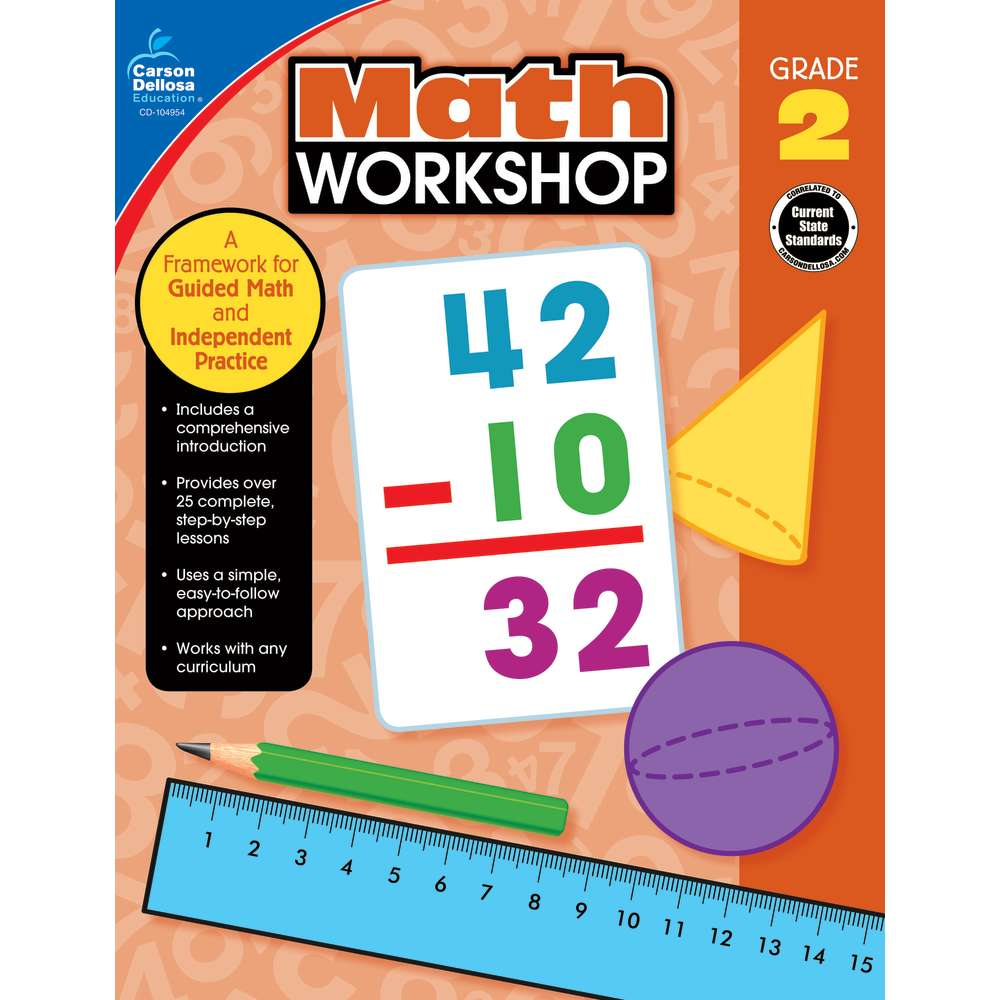•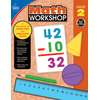•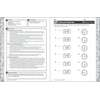•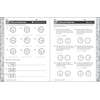•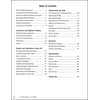### Product Media

Product Number: CD-104954
\$16.99
Enhance your curriculum and implement the math workshop method with this teacher resource for second grade.Math Workshop for ...
Quantity

Enhance your curriculum and implement the math workshop method with this teacher resource for second grade.

Math Workshop for second grade provides complete small-group math instruction for these important topics:

• arrays
• skip counting
• measuring length

Simple and easy-to-use, this teacher resource for second grade math teachers complements any curriculum.

Like reading and writing workshops, math workshop is an instructional model that combines whole-group lessons with leveled guided math groups and independent practice. It allows teachers to give students direct, leveled instruction while providing opportunities for practice and skill review. Math Workshop for second grade simplifies the workshop method with a comprehensive introduction and over 25 step-by-step lessons. This teacher resource for second grade math also includes these helpful features:

• comprehensive lesson plans
• leveled practice pages
• hands-on activities for every lesson

The Math Workshop series for kindergarten through fifth grades gives teachers everything they need to implement the math workshop method. Each book contains 28 complete lessons, a thorough introduction, and reproducible game templates. Each lesson begins with an essential question, a warm-up activity, and a whole-group lesson. It is followed by three leveled small-group lessons and a short assessment. Lessons are rounded out with a practice worksheet for each small group and an activity to practice the skill. Teachers are also provided with math talk questions and a math journal prompt to extend learning. The Math Workshop series gives teachers the flexible tools needed to begin small-group math instruction.

CD-104954